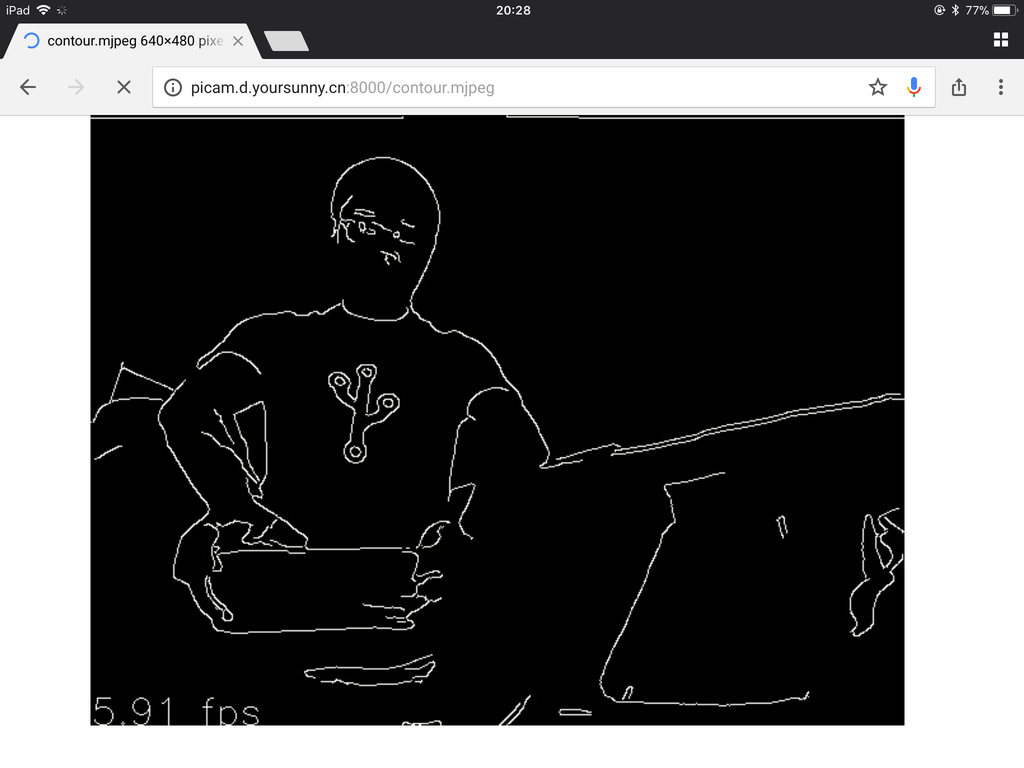# GPU Accelerated Contour Detection on PiCamera

Earlier this month, I spent a week building OpenCV 3.2.0, with the intention to reproduce the contour detection demo I witnessed at MoCoMakers meetup. I successfully made contour detection working on PiCamera through MJPEG streaming. P.S. Can you tell the Hack Arizona 2016 shirt?## How MocoMakers's Demo Works

``````def makeContour(image):
gray = cv2.cvtColor(image, cv2.COLOR_BGR2GRAY)
gray = cv2.GaussianBlur(gray, (3, 3), 0)
edged = auto_canny(gray)

def auto_canny(image, sigma=0.33):
v = np.median(image)
lower = int(max(0, (1.0 - sigma) * v))
upper = int(min(255, (1.0 + sigma) * v))
edged = cv2.Canny(image, lower, upper)
return edged
``````

Their code works with a MJPEG stream from an Android phone. It extracts a JPEG frame from the video stream, processes the image through `makeContour` function, and displays the result. The `makeContour` function converts the RGB image to grayscale, blurs the grayscale image, and runs the Canny Edge Detection algorithm.

## A Naive Translation

I want to have contour effect on my NoIR Camera Module (paid link), instead of an MJPEG stream from another device. PiCamera is the official Python library to work with Raspberry Pi cameras, and it can capture still images and videos in various formats. There is even an example of capturing a picture to an OpenCV object.

It was straightforward to stitch the code together. This code initializes the camera, captures image frames, and processes them with the same logic as `makeContour` and `auto_canny`. Since my Raspberry Pi Zero W (paid link) isn't connected to a monitor, instead of displaying locally, I'm streaming the output as MJPEG over HTTP to another computer.

``````def handleContourMjpeg(self):
import cv2
import numpy as np
width, height, blur, sigma = 640, 480, 2, 0.33
self.mjpegBegin()
with PiCamera() as camera:
camera.resolution = (width, height)
bgr = np.empty((int(width * height * 3),), dtype=np.uint8)
for x in camera.capture_continuous(bgr, format='bgr'):
image = bgr.reshape((height, width, 3))
image = cv2.cvtColor(image, cv2.COLOR_BGR2GRAY)
image = cv2.GaussianBlur(image, (3, 3), 0)
v = np.median(image)
lower = int(max(0, (1.0 - sigma) * v))
upper = int(min(255, (1.0 + sigma) * v))
image = cv2.Canny(image, lower, upper)
self.wfile.write(cv2.imencode('.jpg', image))
self.mjpegEndFrame()
``````

I did see the cool contour effect of my muscular body, but the frame rate was low: I got only 1.6 Frames Per Second (FPS). 1.6 FPS is not a satisfying experience, so I started optimizing the code.

## Optimizing the Code: Video Port

`camera.capture_continuous` by default captures still images using the "image port" of the Pi camera. For rapid capturing, I added `use_video_port=True` argument to this function invocation. It asks the camera to capture a still image via the "video port", which should enable higher FPS at the expense of lower picture quality.

The performance improved to 2.6 FPS after this change.

## Optimizing the Code: YUV

The first step of `makeContour` is converting the "bgr"-format image to grayscale. "bgr" stands for "blue-green-red", which is one of the output formats supported by PiCamera. Can I eliminate this step, and have PiCamera provide a grayscale image directly?

I looked through the list of supported formats, but did not find a grayscale format. However, there is a "yuv" format, where Y stands for "luminance" or "brightness". On the other hand, a grayscale image can be the result of measuring the intensity of light at each pixel. Bingo! I just need to extract the "Y" component of a YUV image, and get a grayscale image.

Where's the "Y" component? PiCamera docs give a detailed description: for a 640x480 image, first 640x480 bytes of the array would be "Y", followed by 640x480/4 bytes of "U" and 640x480/4 bytes of "V". Therefore, I can extract "Y" component into `image` variable like this:

``````yuv = np.empty((int(width * height * 1.5),), dtype=np.uint8)
for x in camera.capture_continuous(yuv, format='yuv', use_video_port=True):
image = yuv[:width*height].reshape((height, width))
``````

This change brought the most significant performance improvement: the contour camera is running at 4.7 FPS.

## Optimizing the Code: Blurring in GPU

The second step of `makeContour` is a Gaussian blur filter. Luckily, PiCamera gives a way to do this in the GPU:

``````camera.video_denoise = False
camera.image_effect = 'blur'
camera.image_effect_params = (2,)
``````

Although the GPU's blurring effect is not exactly same as `cv2.GaussianBlur`, the contour still looks awesome. The performance reached 4.9 FPS (for the same scene as other tests that is more complex than the screenshot at the beginning of this article).

## The Complete Code

``````#!/usr/bin/python3
import time
from http.server import HTTPServer, BaseHTTPRequestHandler
from picamera import PiCamera

class MjpegMixin:
"""
Add MJPEG features to a subclass of BaseHTTPRequestHandler.
"""

mjpegBound = 'eb4154aac1c9ee636b8a6f5622176d1fbc08d382ee161bbd42e8483808c684b6'
frameBegin = 'Content-Type: image/jpeg\n\n'.encode('ascii')
frameBound = ('\n--' + mjpegBound + '\n').encode('ascii') + frameBegin

def mjpegBegin(self):
self.send_response(200)
'multipart/x-mixed-replace;boundary=' + MjpegMixin.mjpegBound)
self.wfile.write(MjpegMixin.frameBegin)

def mjpegEndFrame(self):
self.wfile.write(MjpegMixin.frameBound)

class SmoothedFpsCalculator:
"""
Provide smoothed frame per second calculation.
"""

def __init__(self, alpha=0.1):
self.t = time.time()
self.alpha = alpha
self.sfps = None

def __call__(self):
t = time.time()
d = t - self.t
self.t = t
fps = 1.0 / d
if self.sfps is None:
self.sfps = fps
else:
self.sfps = fps * self.alpha + self.sfps * (1.0 - self.alpha)
return self.sfps

class Handler(BaseHTTPRequestHandler, MjpegMixin):
def do_GET(self):
if self.path == '/contour.mjpeg':
self.handleContourMjpeg()
else:
self.send_response(404)

def handleContourMjpeg(self):
import cv2
import numpy as np
width, height, blur, sigma = 640, 480, 2, 0.33
fpsFont, fpsXY = cv2.FONT_HERSHEY_SIMPLEX, (0, height-1)
self.mjpegBegin()
with PiCamera() as camera:
camera.resolution = (width, height)
camera.video_denoise = False
camera.image_effect = 'blur'
camera.image_effect_params = (blur,)
yuv = np.empty((int(width * height * 1.5),), dtype=np.uint8)
sfps = SmoothedFpsCalculator()
for x in camera.capture_continuous(yuv, format='yuv', use_video_port=True):
image = yuv[:width*height].reshape((height, width))
v = np.median(image)
lower = int(max(0, (1.0 - sigma) * v))
upper = int(min(255, (1.0 + sigma) * v))
image = cv2.Canny(image, lower, upper)
cv2.putText(image, '%0.2f fps' %
sfps(), fpsXY, fpsFont, 1.0, 255)
self.wfile.write(cv2.imencode('.jpg', image))
self.mjpegEndFrame()

def run(port=8000):
httpd = HTTPServer(('', port), Handler)
httpd.serve_forever()

if __name__ == '__main__':
import argparse
parser = argparse.ArgumentParser(description='HTTP streaming camera.')